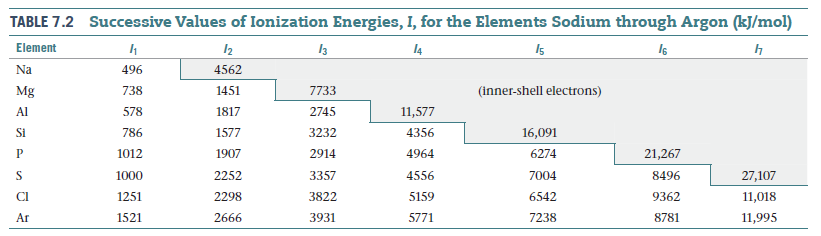# Problem: Construct a Born-Haber cycle for the formation of the hypothetical compound NaCl2, where the sodium ion has a 2+ charge (the 2nd ionization energy for sodium is given in Table 7.2 in the textbook).How large would the lattice energy need to be for the formation of NaCl2 to be exothermic?

###### FREE Expert Solution

We’re being asked to calculate the lattice energy of NaCl2 needed for the reaction to be exothermic.

Recall that lattice energy is the energy required to combine two gaseous ions into a solid ionic compound:

Mx+(g) + Ny–(g)  MyNx(s)

To calculate for lattice energy, we need to do the Born-Haber cycle for NaCl2

Na(s) + Cl2(g)  NaCl2(s), ΔH˚f

97% (151 ratings)###### Problem Details

Construct a Born-Haber cycle for the formation of the hypothetical compound NaCl2, where the sodium ion has a 2+ charge (the 2nd ionization energy for sodium is given in Table 7.2 in the textbook).

How large would the lattice energy need to be for the formation of NaCl2 to be exothermic?